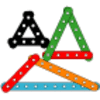#### You may also like### I'm Eight

Find a great variety of ways of asking questions which make 8.### Let's Investigate Triangles

Vincent and Tara are making triangles with the class construction set. They have a pile of strips of different lengths. How many different triangles can they make?### Noah

Noah saw 12 legs walk by into the Ark. How many creatures did he see?

# Getting the Balance

##### Age 5 to 7 Challenge Level:

Daniel from Englefield Infant School had a go at this challenge. First he tried 8 and 3 on the left, as the question suggested:

8+3=1+2+8
8+3=1+3+7
8+3=1+4+6
8+3=2+3+6
8+3=2+4+5

I like the way you have written these in a very logical order, Daniel. Well done! Next, Daniel tried 10 and 9 on the left side of the balance:

10+9=1+8+10
10+9=2+7+10
10+9=2+8+9
10+9=3+6+10
10+9=3+7+9
10+9=4+5+10
10+9=4+6+9
10+9=4+7+8
10+9=5+6+8

Fantastic! Izzy from Tonbridge Grammar School reminds us that:

Whatever the sum of numbers of weights on one side add up to has to be equal with the mass of weights on the other.

Thank you to you both.